In Part 1, we learned what a fraction is, the parts of a fraction, and how to add, subtract, divide and multiply fractions! But all of the fractions we worked with were proper fractions. Today, we're going to work with improper fractions.

What are 'Improper' Fractions?

Now, what even is an improper fraction? Did they forget to keep their pinkies up while they sipped their tea?

Jokes aside, improper fractions are quite easy to spot. If the numerator is bigger than the denominator, the fraction is improper!Why? Well, for a fraction to be 'proper' it must be part of a whole -- meaning, it must be less than or equal to the whole thing (ex: if you have a pizza pie with 8 slices, the numerator must be 8, 7, 6, 5, 4, 3, 2, or 1. How could you have more slices than what's available in the whole pie?)

Well, an improper fractions is precisely that! Think of it in terms of pizza night. Your parents ordered one whole pie, but it just wasn't enough. So they order one more whole pizza. What does that mean? Well, you have more than one whole pie!Who knew improper fractions could be so yummy?

Now, imagine from the 16/8s, your brother eats 3 slices. How many slices are left? Well, we just need to subtract 3/8s from 16/8:Voilà! Easy as pie :)

What is a Mixed Number?

Have you ever heard someone say “I have 13 8ths of a pizza left?” Probably not. That's a really weird way to talk. Rather (if they were math affinados), they would say something like "I have one and 5/8s of a pie left!"

This way of expressing fractions is called a mixed number. A mixed number is just a whole number plus a fraction! So write how much pizza is left after your brother ate 3 slices as a mixed number, we would write 1 + 5/8s, or: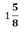Remember, mixed numbers cannot be considered whole numbers because part of the number is still a fraction! Mixed fractions are just whole numbers plus a lil' extra :)

Converting Mixed Numbers to Improper Fractions

Converting a mixed number into an improper fraction is quite simple.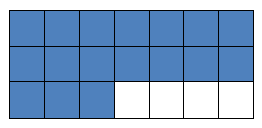If we had for a mixed number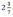and we need to make this an improper fraction....

1. Multiply the Denominator * the Whole Number
2*7 = 14

14+3 = 17

3. Place the sum on-top of your original denominator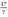Ta da! You just converted a mixed number into an improper fraction!

Converting Improper Fractions to Mixed NumbersIf we had the improper fraction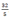and we need make this into a mixed number...

1. Divide the numerator by the denominator
(most of time times there will be a remainder)
32 / 5 = 6 R 2.

2. Your quotient becomes the whole number,
& the denominator stays the same!
So,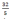=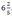Whether proper or improper or even mixed, fractions actually aren't that hard! Your child will just need a thorough explanation of the concepts and lots of practice. Because, remember, even as your child gets older, fractions will always be there in some shape or form (both in their studies, and in real life).

Therefore, becoming familiar with fractions at an early age and, more importantly, becoming comfortable with them in any shape or form can give huge boost in confidence and aptitude! So try and teach your child, they have all the power and knowledge necessary to defeat fractions. They just need to try!

1

## What To Do Next…

1 Get your free 60-minute Child Assessment and learn:

• If your child is learning at the appropriate age level.